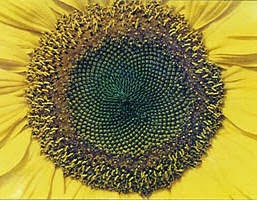## Monday, March 9, 2015

### Plants Don't Do Math

There's no evidence that plants have any kind of higher-order cognitive abilities. They can't really perform calculations. So why do so many of them exhibit Fibonacci's sequence in their leaf arrangements, florets, etc.? Old Fib's sequence also provides us with a good way to estimate the Golden Ratio, which is an irrational number. And the Golden Spiral that is made using the Golden Ratio also appears in sunflower seed arrangements, as well as the shells of marine animals.

What's going on?

It turns out that these arrangements in nature seem to be the best ways to maximize space. The Golden Spiral is the best way to pack the most seeds into the head of the sunflower, for example.Golden Spirals in a sunflower
To estimate the Golden Ratio using the Fibonacci Sequence, just take any two of the adjacent numbers and divide the larger by the smaller. It works better with the larger numbers. Take 6765 divided by 4181 (the 21st and 20th numbers), for example. That equals 1.618034, which is a pretty close estimate. Humans love the Golden Ratio - it seems to touch on something deep within our brains. It shows up all the time in architecture and art - sometimes not intentionally.

Fish scales, however, seem to be arranged randomly. So fish aren't that cool. I thought that I read years ago how fish scales are arranged in a way related to Fibonacci's Sequence, which is why this subject is tied to today's comic (which includes the trout again). However, a search on the subject turned up no evidence and I have to conclude that either my memory is faulty or my information was bad. Probably my memory.

But since we're here, if you'd like to calculate the first n Fibonacci numbers or the nth number in the sequence, you can use a recursive function. I wrote this one a while back in Java. It takes a number n as a command-line argument and prints out, one per line, the first n numbers in the sequence.

```public class Fibonacci {

/**
* Calculate the (n+1)th number in the Fibonacci Sequence.
* For example, 0 will give you the first number (0), 1 the
* second number (1), 2 the third number (1), and so on.
*
* @param n the sequence number
* @return the value of that position in the sequence
*/
private static int fib(int n) {

if (n == 0) {
return 0;
} else if (n == 1) {
return 1;
} else {
return fib(n-1) + fib(n-2);
}

}

public static void main(String[] args) {

if (args.length < 1) {
System.out.println("no arguments given");
System.exit(1);
} else {

int f = 0;
try {
f = Integer.parseInt(args);
} catch (NumberFormatException nfe) {
System.out.println(args + " is not an integer");
System.exit(1);
}

for(int i = 0; i < f; i++) {
System.out.println(fib(i));
}

}
}
}
```

The function is really simple, since the method of calculation is so simple. The first number in the sequence is 0 and the second number is 1. So there are two cases to end the recursion. For all others, the function just calls itself for the previous 2 numbers and adds them together. I love recursion. And frogs.Amphibian.com comic for 9 March 2015